# Voltage Control and the Analog Synthesizer - from Experimental Television Center Studio System Manual

 Publication Type Unpublished Authors Brewster, Richard Source (1978) Keywords tool-text
Full Text:

The Center's Analog Synthesizer is a modular system for creating different types of changing voltages. The final outputs of the Synthesizer will be used in two distinct ways:
1. as control signals for the image processing modules
2. as synthesized audio for sound tracts

INTRODUCTION TO VOLTAGE CONTROL
The system has been designed specifically to produce slowly changing voltages suited to controlling various parameters on the imaging modules such as fading, keying, colorization and so on. One way to view the Analog synthesizer is as a machine that combines various simple changes (represented by changing voltages, which are usually periodic in nature) for the purpose of obtaining interesting and complex changes in imagery. Our model has been the modular electronic music synthesizer and we have retained many specific standards from that industry. The Analog Synthesizer is compatible with most commercially made audio synthesis equipment, so you are encouraged to bring your own synthesizers to the studio if you wish. If you plan to do so. please check first with the Center's staff to be sure that the appropriate cables are available.

To use this machine you will have to think about voltage control. We can think of voltage as a quantity that can be measured discretely, for example a given number of volts, 5V. For this to be meaningful we need a point of reference. Usually ground (earth) potential is used as a reference as 0V. All sources of constant voltage (DC which stands for direct current) are signed. They have a positive and negative terminal, like a battery. If the negative terminal were connected to ground, then our 5V source will have +5V with respect to ground at its positive terminal. If the positive terminal were connected to ground instead, then we would have -5V at the negative terminal with respect to ground. In the Analog Synthesizer we use both polarities of voltage with respect to ground and the term "bias' to describe this relationship.

Our control voltage standard is -5V to +5V. Both the Analog Synthesizer and the video image processing modules have been built to respond to this voltage range. This means that a certain aspect of a module's output will be varied from one useful extreme to the other by a sweep of -5V to +5V. This range can be expressed as 10V peak to peak, abbreviated as 10V P-p. For example: in controlling video pedestal, -5V at the control input would produce black while +5V would yield a white pedestal level, with values in between producing various grey levels. You will find that the useful range will lie somewhat within the -5V to +5V standard. So it will take less than 10V P-p (peak to peak) to sweep the effective range. Since the Analog Synthesizer puts out 10V P-p, it is a simple matter to reduce this voltage to the desired Ievel .

Most of the control knobs on the video modules have associated jacks for taking in control voltages. A changing control voltage applied to one of these jacks has the effect of "automatically" turning the knob. Advantages of using a control voltage instead of just turning the knob include (1) variations which are smoother or quicker than would be possible by hand, (2) increased precision and (3) step-like movement. Manual control is useful for complex changes.

There are three important aspects of a control voltage:
1. GAIN the total voltage excursion, expressed in volts peak to peak
2. BIAS the relative placement of the waveform relative to ground
3. WAVESHAPE the nature of chance with respect to time

We represent these aspects graphically in the example below by plotting voltage (expressed along the Y axis) with respect to time (expressed along the X axis).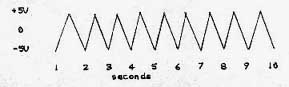In this example, the GAIN is 10V P-p, the BIAS is centered at ground or OV, and the WAVESHAPE is called triangle. The rate or FREQUENCY of this signal is 1 Hertz expressed as 1 Hz. This means that the waveshape takes one second of time to complete its form and begin to repeat itself. A triangle waveform is called PERIODIC because it repeats itself on a regular basis within a given period of time. Hertz (Hz) refers to the number of cycles the waveform goes through in one second.

To illustrate how such a control signal can be used in the video synthesizer. let's take this example signal and look at how the patch would be set up.The attenuator, which means reducer, is simply a potentiometer (pot) or knob that enables us to lower the gain of a signal with respect to ground. An ordinary volume control is an application of this type of device. In the patch, Point A is the example signal from the Analog Synthesizer. This is patched to the input of the attenuator. Depending on the knob setting, we can obtain any peak to peak value less than 10 V P-p at the output of the attenuator. The graph "Signal at Point B" shows the attenuator output, arbitrarily selected to be 2V P-p. This is the signal we will apply to a control input on a video module. Here we must examine how the control knob on the video module interacts with the incoming control signal.

The first point to understand is that the knob is also a source of a control voltage. If there is no input to the VC input jack, then the knob voltage is the only one used. When a voltage is applied to the VC input, it is mixed with the knob voltage. Since it is the nature of our mixers to invert (change the sign) of the incoming voltage. the knobs have been made so that counter-clockwise represents +5V and clockwise represents -5V This is so that after the mixer, the voltage will be positive for clockwise-of-center rotations. Thus turning the knob clockwise results in black to white, low to high and so on. This also means that, with the knob centered at 0V, a control input of +5V is the same as having the knob all the way counter-clockwise with no control input. You must think of a falling (negative going) control signal as producing the effect of turning the knob clockwise.

Bellow is a graph of the voltage output from a knob. The following graph represents an inverted mix of this knob voltage and our attenuated control signal. You can see how the relative placement of the control signal is moved by the knob. This is called BIASING the signal.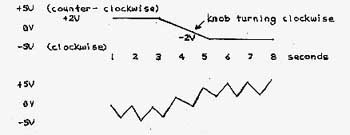Let's continue to analyze how the attenuator and bias knobs allow us to get the effect we want. As an example, let's control the clip of a keyer. With no control voltage input, the extremes that we wish are at these knob settings: 11 o'clock and 2 o'clock which are about one-third of the total knob rotation. From this we can guess that we will need about 3 or 4 volts P-pk to get the entire sweep we require. As Iong as we start with a larger signal, the attenuator will allow us to achieve the proper gain. With the signal applied to the VC input, the control knob can now be used to bias the signal so that the start point and end point of the effect are where we want them. At this point it is a matter of adjusting the knobs while observing the image in order to set up the desired effect.

Now we will look at the different types of signals in the Analog Synthesizer. A signal can most generally be defined as a voltage that changes with respect to time. In the Analog Synthesizer we can think of a signal as the changing voltage of an oscillator that we are going to process and use as an input to video image processing modules. The terms signal and control signal are relative and refer only to use. In general, a control is lower in frequency than -the signal it is modifying.

In the Analog Synthesizer signals are classified according to bias, function and waveshape.

BIAS
1) +- signal This type of signal usually remains within +-5V. However if several of these are added in a mixer, the total possible range is about +-12V. This is the maximum voltage excursion in the Analog Synthesizer.
2) + signal This type never goes negative and usually stays in the 0 to +5V range. Certain modules output this type of signal and if a negative signal is presented at their inputs it will not pass through. The output will hold at 0 V.

FUNCTION
1) PULSE This is sometimes called TRIGGER. It is a short positive voltage excursion from 0 to +10V and back. It is the point in time that the voltage jumps high that is significant. This point may be used to determine the start of other events in the system.
2) GATE A signal which is either O V or +5V. O V signifies OFF while +5V signifies ON. This acts like a switch to control the duration of an event, or how Iona it goes on.

WAVESHAPE
Signals classified according to waveshape are named for their graphic representations. See the following page. These periodic waveforms repeat. The number of periods in one second is the frequency in Hertz. The terms positive and negative ramp refer to direction of change, not to bias. Note that inverting (turning upside-down) a ramp or pulse changes the shape, while inverting a triangle, sine or square does not.

These are commonly used waveforms. The XY axis represents voltage. The XY axis represents time.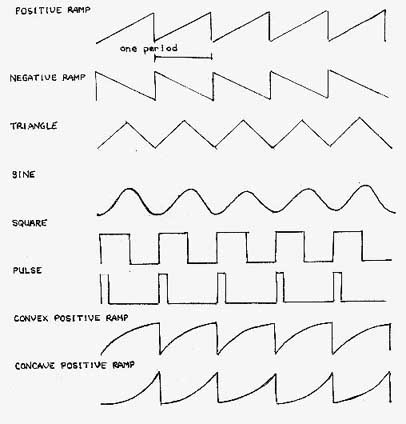DETAILED MODULE DESCRIPTION
The Analog Synthesizer has two boxes each with its own set of modules. The panel controls are listed first and then the functions are described. Module panels have the following features: 1.Signal inputs 2. Control inputs 3. one or more outputs 4. knobs for setting initial points (bias knobs) 5. knobs for inverting or attenuating control voltages and 6. special devices such as switches or lights. Inputs are generally to the left and top while outputs are to the right and bottom. Black lines between jacks indicate internal electrical connections.

NEGATIVE RAMP
RATE        Bias pot setting initial rate of fall
VC              Attenuator/inverter pot with control voltage input
IN               Main signal input
OUT           Main signal output
P Pulse      Output
LAMP       Output level indicator

The function of this module is to limit the rate at which the output can follow as the input voltage falls. This rate is initially set by the RATE bias control, with more cIockwise settings corresponding to higher rates. The rate may also be varied by a control voltage applied at VC. The knob above VC attenuates and/or inverts the control voltage in this manner. At the center of its rotation, an incoming control voltage will be most greatly attenuated, that is will have the Ieast effect. Turning the knob to the right, clockwise, will cause a positive voltage being presented at the VC input to effect a higher rate. Counter-clockwise rotation with a positive VC input will lower the rate. The farther the knob is turned in either direction, the greater the effect. Negative voltages may be applied to the VC input.

The negative ramp module puts out only positive voltages, thus the input must be positive to pass through. There are two outputs. The main signal output will accurately follow a rising voltage at the input, while limiting the rate of a falling input. The pulse output remains at +10V while the main output is close to 0V. If the main output rises above about .2V, the pulse output will go OV. The lamp indicates main output voltage, getting brighter as the main output increases in voltage. If the output frequency is rapid, the light will appear to remain constant At a medium brightness.

What happens if the pulse output is patched to the main signal input? First, the output has been at 0, so the pulse output is high, +10V. When the connection is made the main output rises quickly following the rising input voltage, but as soon as the main output rises this causes the pulse output to go to OV. Since the main output can't fall any faster than the control will allow, we will get a falling ramp at the main output. When the ramp approaches 0 V the pulse output jumps high and the process is repeated. Thus we have an oscillator with ramp and pulse outputs.

When patched as above, the two negative ramp modules on the Analog Synthesizer each have different frequency ranges. These are shown below.

MODULE                                 RANGE                              with CONTROL VOLTAGE
Top Negative Ramp         38 sec/cycle to 500 Hz              up to 1200 Hz
Bottom Neg. Ramp          17 sec/cycle to 1000 Hz            up to 2500 Hz
Top Positive Ramp           23 sec/cycle to 1000 Hz            up to 1700 Hz
Bottom Pos. Ramp            7 sec/cycle to 2000 Hz             up to 4000 Hz
Top Triangle                 120 sec/cycle to 1700 Hz
Bottom Triangle              90 sec/cycle to 2000 Hz

POSITIVE RAMP
RATE            Bias pot setting initial rate of rise
VC                 Attenuator/invertor pot with control voltage input
IN                 Main signal input
OUT             Main signal output (TOP)
P1                 Pulse output one
P2                 Pulse output two
START         Input for starting ramp
SUS              Input for starting ramp and sustaining at +5V
Switch           Performs gate function for sustain
VC                Voltage control of waveshape from lower output
OUT              Sine type waveform output (BOTTOM)

The function of this module is to limit the rate at which the output can follow as the input voltage rises. This rate is controlled in a manner similar to the negative ramp. There are four outputs. The main signal output limits the rate of a rising input, while accurately following a falling input. P1 is low while the ramp is above 4.5V and goes high at the point the ramp wave falls. There must be an input to Start or Sus to get an output from P2. P2 stays high while the ramp is rising and holds at OV if the main output is also at OV. For both pulse outputs high equals +5V. Like the negative ramp, an oscillator can be patched by connecting PI to IN. The resulting ramp wave at the main output is about 4V P-P from +.5V to +4.5V. A sine-type waveform is simultaneously available at the lower output, and its shape is voltage controllable. This output is also positively biased and has a gain of about 2V .

An oscillator can also be created by patching P1 to Start or Sus. This will give an output at P2 as well as raising the main signal output gain to 5V P-p.

A rising ramp may be started by supplying a trigger pulse to the Start input. When this ramp is completed and returns to 0V, it will remain at 0V until another trigger is received. A trigger applied to Sustain will also start a ramp. If a gate is applied to Sustain, a ramp will be initiated also, and if the gate is still present when the ramp has reached its maximum, the main output will hold at +5.5V until the gate is removed. A gate patched to Start will perform the starting function, but will not sustain the output.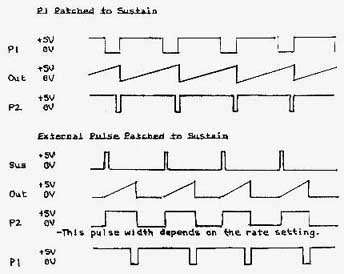WAVSEHAPE (WS)
GAIN             Input attenuator
SHAPE          Bias pot setting initial wabeshape
VC                Control voltage input #1
VC                Control voltage input #2
IN                 Main signal input
OUT             Main signal output

The purpose of this module is normally to convert a positive ramp wave into a sine-type wave with a voltage controllable waveshape. For proper operation in this mode the input should be a positively biased positive ramp wave (such as the output of a positive ramp) with a gain of about 2V P-p. An input attenuator is provided for setting this gain.

The shape control is a bias pot which sets the initial waveshape. Centered, the output is an approximate sine wave. Varying the control results in a more ramp-like waveform at the output. The extremes of rotation produce nearly concave positive or negative ramps. The output is positively biased with a gain of about 5V P-p.

The VC input directly below the shape control allows voltage control similar to the shape control pot. With the pot centered, +-5V is sufficient to sweep the pot range. The lower VC input hats the effect of biasing the output so as to cut off the lower portion of the signal, which also changes the waveshape.

Other waveforms may be applied to the input. Note that at audio frequencies the WS is used as a timbre modifier.

INVERTER X2
This module which has no controls, is designed to convert a 0 to +5V signal to a +-5V signal. Since it also inverts, the in/out relationship looks like this: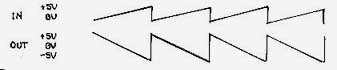You can see that an input of 0V (no input) will Ieave the output at +5V. +2.5V in comes out OV. +5V in comes out -5V. The gain is doubled. A hint: If you want to invert but leave the output still at OV to +5V, simply attenuate the input to 2.51%). Note that a negatively biased input will cause the output to go above +5V. This module is meant to increase the gain available from the Ramp modules. Since the output from the Triangle patched to oscillate is -1V to +4V, passing this signal through Invert X2 will give +7V to -3V out. This is still within a usable range.

RANDOM
WH ITE 1      White noise output with energy concentration in center
WHITE 2       White noise output with energy concentration a t edges
PINK             Pink noise output filtered from WHITE 1
RAMP +        Fast random ramp output, positive bias, 5V P-p
RAMP +-      Fast random output. +-bias, 10 V P-p
SAMPLE       Button changes Step outputs to new random level
SAMPLE       Jack accepts trigger pulse input for above function
STEP +          Random held voltage output, + bias
STEP +-        Random held voltage output, +- bias
TREM           7 Hz sinewave output with random amplitude
Q                  Varied randomness is TREM output
PULSE          Random pulse output
RATE           Varies range of random pulse frequency

The random module is. a. source of different kinds of randomly changing voltaqes. Random sources are categorized by their average rates of change. White noise contains the widest range of frequency variations: the entire audio range. 20 Hz to 20 KHz. Pink noise contains less high-frequencies than white noise, while the random ramps are low in frequency. Patching these sources to an audio monitor will give you a good idea of this. The step Outputs have a rate of change equal to the sample pulse rate.

White 1 output is biased about ground and is generally 5V P-p. The probability for an instantaneous output is higher for values nearer to ground. White 2 is 8V P-p biased at ground with greater probability of an instantaneous output being at either + or -4V.

The Step outputs are from a sample- and- hold- connected internally to a random source. Pushing the button or supplying a pulse to Sample (the only input on the module) will set the Step outputs to new random levels. These voltages drift slightly.

Patching the random tremolo output to an oscillator VC input will quickly show how this functions. Notice a time lag after turning the Q knob before the effect changes.

The Rate control adjusts the random pulse output from a pulse every few seconds to dozens per second. When setting this control allows 15 seconds or so to observe the effect at one setting.

ONE SHOT
S                Sample Input
T                Track input
OUT          One shot output

This module has no controls. Normally the output sits at +10V. If a trigger pulse is supplied to the S input, the output will fall to 0V for a preset time interval of about I ms, then rise quickly back to +10V. A gate to the T input will cause the output to hold at 0V as long as the gate is present.

Patching the output of this module to the Hold input of the Triangle module creates a sample and hold. During this patch, a gate to the Track input returns the Triangle to its following mode.

ONE PULSE
This module puts out one 100 microsecond pulse each time the button is pushed. Use this +or a manual trigger.

GATE
This switch when up applies +6V to its output jack and OV (ground) when down. Use this for a manual gate (patch to Hold. Track, Sustain, etc.) .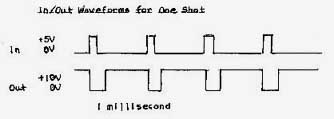TRIANGLE
RATE         Bias got setting initial triangle period
VC             Attenuator got with control voltage input
IN              Main Signal input
OUT          Main signal output
P                Pulse output (+-11V, 22V P-p)
H               Hold input
Lamp         Output level indicator

The triangle module limits the rate at which the output can follow both rising and failing voltages at the input. Unlike the Ramp modules, the -Triangle inputs and outputs may be biased positive or negative. The main and pulse outputs are related in this manner: as the main output falls below -IV, the pulse output goes to +IIV and holds there until the main output rises above +4V. The pulse output then falls and holds at -IIV until such time as the main output again falls under -IV. Patching the pulse output to IN Yields an oscillator with the following waveforms:Another difference from the Ramp modules is that the VC attenuator pot does not invert an incoming control voltage: positive voltages always increase the rate.

The Hold input accepts a +5V or higher gate signal. When the gate is presented the main output will hold at the voltage it had at the moment the gate went high. This same function may also be accomplished by bringing the VC input to a sufficiently negative value, depending on the initial rate setting.

The lamp is an output level indicator which functions similarly to the lamp on the negative ramp module.

ANALOG SYSTEM MODULES IN BOX 2

VOLTAGE OSCILLATOR (VCO)
FREQ        Bias pot setting initial frequency, wide range
FINE         Bias pot setting initial frequency narrow range
VC            Most sensitive control input, with attenuator
LIN           Least sensitive control input, inverted
1V/OCT   Control input set for I V increase to double frequency
HI/LO      Audio/subaudio range selector
H/EXT/V  Sync selector switch
EXT          Sync input
RAMP      Sawtooth waveform output
TRI           Triangle waveform output
SIN          Sine waveform output

This module is a music quality oscillator. Its function is to generate periodic waveforms of three shapes, the controlled parameter being the frequency of the outputs. There are a number of control inputs which may be used simultaneously. The outputs are all the same frequency and are all similarly affected by the controls. A HI/LO switch determines the mode of operation. HI mode is that of an audio range oscillator. LO mode is that of a very low frequency control oscillator.

In LO range the frequency is manually variable by the bias pots from I cycle every 20 seconds to about 15 Hz. In HI the manual range is 16 Hz to 16 KHz. Both of these ranges can be expanded with voltage control. Negative control voltage will lower the LO range infinitely until oscillation stops. Positive control voltage can extend the HI range up to 100 KHz. however at this frequency the outputs are degraded in amplitude and shape.

All FM (Frequency Modulation) inputs allow exponential control of the frequency. This means that for an increase of a set amount of voltage (for example. IV) the frequency will be multiplied by some factor. In the case of the V per octave inputs. a IV increase will double the frequency. The VC input with attenuator allows adjustment of this factor. and at high settings the frequency may be multiplied by a factor of 4 or more for each volt increase of control voltage. The LIN control input is much less sensitive, and operates inversely: an increase of control voltage lowers the frequency. This ratio is set at approximately 6V/octave.

Sync options are selected with the sync switch. Center is off, while H/EXT normally supplies horizontal sync for use at high frequencies (above 15 KHz). Should a signal be patched to the EXT sync input, this input is selected instead of horizontal sync. Signals patched to EXT should have a sharply rising edge for proper syncing. The Y position selects vertical sync. Standard video sync must be plugged to the BNC inputs at the left of the panel for these functions to be used.

All outputs are standard +-5V signals. The phase (relative changes) of the outputs are shown below.COMPARATORS
Under the heading of Comparators are three small modules above the Comparator modules. These will be described first.

1) Capacitor: A patchable capacitor is available between the two jacks containing the symbol for capacitance ( -ll- ). Either jack may be the input. Its function is to block the offset bias of an audio frequency signal. so that the output is rebiased around ground.
2) V and H Vertical and horizontal sync pulses are available at these jacks, pulses going from zero to +8Y for
system compatibility. Example: Start a ramp with a V pulse.
3) Invert A standard analog inverter is made available for changing the sign of the input voltage.

Comparator Panel
IN              Comparator input
OUT          Comparator output
VC            Control voltage input for reference
REF           Bias pot for setting initial reference point.

The function of the comparator is to compare two voltages and signal when one is higher than the other. The output is high 080 if the input is higher than the reference voltage. and zero volts if the input voltage is lower than the reference. Thus if a periodic voltage is applied to the input and regularly crosses the reference point, a pulse wave is the resulting output.

Since the duty cycle of the pulse wave is determined by the relative setting of the reference, this parameter is variable and may be voltage controlled. This is called pulse width modulation. The comparator may be used to obtain pulse or square waves from any oscillating module. Notice that a square wave is a special case of a pulse wave for which the duty cycle is 50%. At audio frequencies pulse width modulation produced interesting timbral changes in the sound. One useful sub-audio application would be to control a VCA. The VCA would switch rapidly from zero to full win. An attenuator on the VCA VC input would be helpful.

Below are the comparator input, reference and output as an example to demonstrate the function: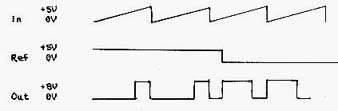MIXERS
1,2,3             Inputs with associated attenuators
4                  "Wild" inputs
- +               Bias pots for setting output offset voltage
OUT           Main output, non-inverting
INVERT     Main output, inverting

The mixers enable a combination of the outputs of two or more modules, with manual control of the mix. All four inputs are added together. This means that if you put the same signal into two of the inputs turned up full, the output will be twice the input. The Wild input simply has no attenuator, This allows the mixers to be patched together for a six-input mixer. Any signals in the Analog Synthesizer may be mixed, but note that up to +-11Y may result. The bias pot allows offsetting which is useful when mixing control voltages.

VOLTAGE CONTROLLED AMPLIFIERS (DUAL VCA)
VC >           Control input for panning and fading
PAN            Bias pot setting initial pan/fade
1,2 GAIN     Bias pots setting individual initial gains
1,2 IN          Respective inputs for VCA 1 and VCA 2
1+2 IN         Input simultaneously to VCA I and VCA 2
1,2 VC         Respective gain control inputs for VCA I and VCA 2
1+2 VC        Simultaneous gain control input for both VCAs
1,2 OUT       Respective VCA outputs
1+2              Sum of the outputs of both VCAs
1-2               Sum of VCA 1 output plus the inversion of VCA 2 output

The VCA provides a means of controlling the gain of a signal by control voltage. Any signal including subaudio, may be processed. This module consists of two independent VCAs with provisions for tandem use in various ways.

Each VCA has: 1) an input for the signal to be gain controlled 2) a control input 3) an output. A bias pot sets the initial gain point, then the gain is increased by positive control voltages and decreased by negative control voltages. With the bias pot set for zero gain, a +5V control signal wiII give unity gain, that is the output will be identical to the input. Control voltages up to + +10V will produce gains of up to two times the input.

Remember that the bias pot adds to the incoming control voltage so that s signal can be doubled by the pot being all the t,-jay in combination with a +5V control input. For instance, if a +-5Y control were used in this case, the output would be varying from zero to two times the input signal amplitude.

The two VCAs are linked in the following ways. First, there is a third control input at the top of the panel for VC panning and fading. This input affects the two VCAs in opposite fashion: as the control voltage increases, VCA I gain increases, while VCA 2 gain decreases. As the bias pot is turned clockwise, the same is true. There is also a fourth control input labeled 1+2 VC. This control input affects both VCAs simultaneously in the same fashion as the individual VC inputs. All inputs may be used -at the same time on this module.

There is also a 1+2 input which sends the signal to be modified to both MCA inputs. This is used during panning. For fading and voltage controlled mixing there are two mixed outputs of the two VCAs. 1+2 is a normal additive mix, while 1-2 is a different mix. Note that with a signal patched to 1+2 ONN, the 1+2 output may be as high as 4 times gain, while the 1-2 output can never be higher than 2 times gain.

When using this module, first center all three pots. This sets the initial gain of both VCAs to .5. Turning the pan pot will cause one VCA to go to unity gain as the other goes to zero gain. The individual bias pots, can be used to balance the overall effect. Leave the pan pot centered for individual use of the VCAs.

For fading the two signals to be cross-faded are patched into inputs 1 and 2 respectively. The output is taken from 1+2 or 1-2 output. For panning, the input goes. to 1+2 IN and the outputs are taken from the respective 1 and 2 outputs.

VOLTAGE CONTROLLED FILTERS (VCF)
HI/LO           Audio/subaudio range selector
GAIN           Attenuator for Main input
Q                  Resonance control (also effects gain of Limit input)
VC               Control input with attenuator/inverter for VCQ
FREQ           Bias pot setting initial filter cutoff frequency
VC               Control input with attenuator/inverter for VC frequency
LIMIT          Signal input limited by 0 setting
MAIN          Main signal input
HP               High pass output
BP               Band pass output
LP               Low Pass output

The function of the VCF is to alter the gain of an input signal with respect to that signal's frequency. The filter cutoff frequency is the reference. For the high pass +unction, input frequencies below the cutoff frequency will be attenuated. The low pass function attenuates frequencies above the cutoff, while the bandpass function attenuates both above and below the cutoff frequency, allowing only frequencies near the cutoff to pass. The 0 function determines the sharpness or closeness to the cutoff frequency, of the attenuating effect.

Since many waveforms contain a complex distribution of different frequencies which determine the timbre of color of a sound, a VCF is often used to modify timbre. Patch a sawtooth wave into the VCF input and manually vary the cutoff frequency. You will notice the common "wah" effect. Listen to each of the outputs and notice the different effects. Notice the action of the 0 control. Patch the signal into the Limit input and notice how the signal is attenuated at high 0 settings. The purpose of this limiting function is to obtain a more constant output level since the filter "peaks", increases its output amplitude sharply at the cutoff frequency. Higher Q settings produce higher peaks, so the limit function is used to counteract this effect.

Both the cutoff frequency and the 0 are voltage controllable, and an attenuator/inverted similar to that on the ramp modules is provided for processing the control voltage coming in.

In LO range, the VCF can be used to modify control voltages. The outputs have a characteristic sinewave pattern, with the BP and LP outputs being 90 degrees out of phase. The BP output leads the LP output by 1/4 cycle. This is different from 1/2 cycle difference produced by a simple inversion. Try patching in a slow ramp and control something with the output.

Finally, the VCF can be made into a VCO by patching the BP output into the Main input. The output will be a sinewave of about +-IOV. The Q and Gain controls must be set fairly high for this to work. Notice that if one of these is decreased slowly, the oscillation dies out gradually, which is sometimes useful.

In this oscillator mode, the HI/LO switch can select audio or subaudio osciIIations.

SUGGESTED PATCHES

1. Output of an oscillator to its own VC input. This will yield the concave and convex waveforms diagrammed earlier.
2. Main out of a + ramp to the main input of a - ramp. Using the switch on the + ramp you can initiate a rising ramp (taken from the - ramp main output) that will hold as long as the switch is up and fall when the switch is down. The rise and fall rates can be set individually.

3. Manual gate to the main input of a triangle module. When the switch goes up the main output will rise at the adjustable rate and hold until the switch is turned down, then fall at the same rate it rose (unless you change the rate control in the meantime)
4. Random pulse output to the sample input of the random module. This will yield random levels at the step outputs at random intervals.
5. Pulse output from a - ramp (patched as an oscillator) to the sample input on the random module. This will yield random voltage levels at the step outputs at regular intervals.